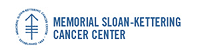## Memorial Sloan-Kettering Cancer Center, Dept. of Epidemiology & Biostatistics Working Paper Series

#### Title

Visualizing Longitudinal Data with Dropouts

#### Abstract

A triangle plot is proposed to display longitudinal data with dropouts. The triangle plot is a tool of data visualization that can also serve as a graphical check for informativeness of the dropout process. There are similarities between the lasagna plot and the triangle plot but the explicit use of dropout time as an axis is an advantage of the triangle plot over the more commonly used graphical strategies for longitudinal data. It is possible to interpret the triangle plot as a trellis plot 1 which gives rise to several extensions such as the triangle histogram and the triangle boxplot. R code is available to streamline the use of the triangle plot in practice.

Biostatistics

COinS